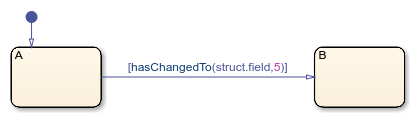# hasChangedTo

Detect change in data to specified value

## Syntax

``tf = hasChangedTo(data,value)``

## Description

example

````tf = hasChangedTo(data,value)` returns `1` (`true`) if the value of `data` is not equal to `value` at the beginning of the previous time step and is equal to `value` at the beginning of the current time step. Otherwise, the operator returns `0` (`false`).```

## Examples

expand all

Transition out of state if any element of `M` has changed value since the last time step or input event and the current value of the matrix `M` is equal to `matrixValue`.

`[hasChangedTo(M,matrixValue)]`Transition out of state if the element in row 1 and column 3 of the matrix `M` has changed to the value `7` since the last time step or input event.

In charts that use MATLAB® as the action language, use:

`[hasChangedTo(M(1,3),7)]`In charts that use C as the action language, use:

`[hasChangedTo(M,7)]`Transition out of state if any field of the structure `struct` has changed value since the last time step or input event and the current value of `struct` is equal to `structValue`.

`[hasChangedTo(struct,structValue)]`Transition out of state if the field `struct.field` has changed to the value `5` since the last time step or input event.

`[hasChangedTo(struct.field,5)]`## Input Arguments

expand all

Stateflow® data, specified as a:

• Scalar

• Matrix or an element of a matrix

• Structure or a field in a structure

• Valid combination of structure fields or matrix elements

If `data` is a matrix, the operator returns `true` when it detects a change in one of the elements of `data`. You can also index elements of a matrix by using numbers or expressions that evaluate to an integer. See Operations for Vectors and Matrices in Stateflow.

If `data` is a structure, the operator returns `true` when it detects a change in one of the fields of `data`. You can also index fields in a structure by using dot notation. See Index and Assign Values to Stateflow Structures.

The argument `data` cannot be a nontrivial expression or a custom code variable.

Standalone charts in MATLAB do not support change detection on an element of a matrix or a field in a structure.

Value of the data at the current time step, specified as the same data type of `data`. `value` must be an expression that resolves to a value that is comparable with `data`:

• If `data` is a scalar, then `value` must resolve to a scalar.

• If `data` is a matrix, then `value` must resolve to a matrix with the same dimensions as `data`.

Alternatively, in a chart that uses C as the action language, `value` can resolve to a scalar value. The chart uses scalar expansion to compare `data` to a matrix whose elements are all equal to the value specified by `value`. See Assign Values to All Elements of a Matrix.

• If `data` is a structure, then `value` must resolve to a structure whose field specification matches `data` exactly.

## Limitations

• Unable to be used within a Stateflow truth table.

## Tips

• If multiple input events occur in the same time step, the `hasChangedTo` operator can detect changes in data value between input events.

• If the chart writes to the data object but does not change the data value, the `hasChangedTo` operator returns `false`.

• The type of Stateflow chart determines the scope of the data supported by the change detection operators:

• Standalone Stateflow charts in MATLAB: `Local` only

• In Simulink® models, charts that use MATLAB as the action language: `Input` only

• In Simulink models, charts that use C as the action language: `Input`, `Output`, `Local`, or ```Data Store Memory```

• In a standalone chart in MATLAB, a change detection operator can detect changes in data specified in a call to the `step` function because these changes occur before the start of the current time step. For example, if `x` is equal to zero, the expression `hasChangedTo(x,1)` returns `true` when you execute the chart `ch` with the command:

`step(ch,x=1);`
In contrast, a change detection operator cannot detect changes in data caused by assignments in state or transition actions in the same time step. Instead, the operator detects the change in value at the start of the next time step.

• In a chart in a Simulink model, if you enable the chart option Initialize Outputs Every Time Chart Wakes Up, using an output as the argument of the `hasChanged` operator always returns `false`. For more information, see Initialize outputs every time chart wakes up.

• When row-major array layout is enabled in charts that use `hasChangedTo`, code generation produces an error. Before generating code in charts that use `hasChangedTo`, enable column-major array layout. See Select Array Layout for Matrices in Generated Code.

## Version History

Introduced in R2007a